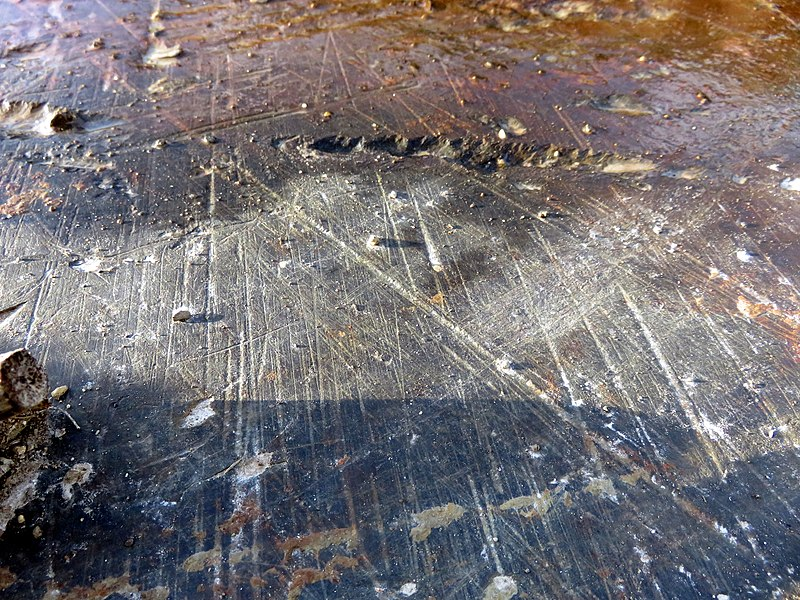# Attenuation of cosmogenic nuclide particles

Davies, B.J., 2022. Dating Glacial Landforms II: Radiometric Techniques, in: Haritashya, U. (Ed.), Treatise in Geomorphology (Second edition). Cryospheric Geomorphology. Elsevier, pp. 249-280. (link)

## Energy decreases as it interacts with matter

The high-energy component of cosmic radiation follows an exponential decrease as a function of the cumulative mass penetrated perpendicular to the surface of the rock (Gosse and Phillips, 2001).

The attenuation length (Λ) is the thickness of a slab of material (rock, air, water, sediment, snow) required to attenuate the intensity of the cosmic-ray flux by a factor of e-1, due to scattering and absorption processes. The attenuation length varies with altitude and latitude, because the geomagnetic field and atmosphere change the energy spectrum (Gosse and Phillips, 2001).

## Attenuation coefficient

The attenuation coefficient is expressed in terms of units of mass length (g cm-2) because the length depends on the total mass traversed and is therefore a function of the material’s density. The attenuation length of solid rock, with a typical bulk density of 2.7 g cm-3, is 121 – 170 g cm-2, and the thickness of rock required to attenuate the cosmic ray flux by a factor of e-1, is 45 to >65 cm. In practice, the attenuation length (Λ) is usually taken to be a constant, at 160 g cm-2 (Balco, 2011; Balco et al., 2008; Gosse and Phillips, 2001).

Length in units of cm can be determined if densities are known and constant (Table 5), by dividing the attenuation length of 160 g cm-2 by the material density.

## Account for sample thickness

When calculating an exposure age, the sample thickness is therefore a crucial parameter because production by spallation within the rock is assumed to have an exponential depth-dependence with a single attenuation length (160 g cm-2) (Balco et al., 2008).

In granite, the spallogenic production rate falls by a factor of two with every ~40 cm of depth, and becomes negligible 2 to 3 m below the surface (Balco, 2011).